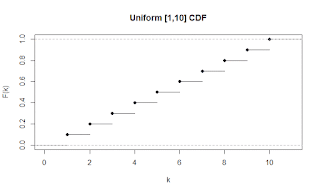## Posts

Showing posts from January, 2015

### Interpolation Search from Any DistributionIn my last blog post , I introduced interpolation search, a searching algorithm that can beat binary search asymptotically. To introduce the concept of interpolation search, I made a simplifying assumption that the underlying data came from a uniform distribution. It is still possible to use interpolation search on non-uniform distributions, as long as you have the CDF for that distribution. Background Info A cumulative distribution function is a function that tells you the probability that a random item from the distribution is less than or equal to a specified value. $$F(k) = P(X \leq k)$$ Thus, $F(k)$ can take on values in the range $[0,1]$ and it is non-descending. For a discrete uniform distribution of integers in the range $[1,10]$, the CDF looks like: And for a continuous normal distribution, the CDF looks like: How to get the CDF There are a number of options to get a handle on the CDF. You can find information online about your distribution, do a one-pass

### Interpolation Search ExplainedIf you have taken an introductory computer science course, you've probably seen the binary search algorithm - an algorithm to efficiently find the index of an item in a sorted array, if it exists. You might not have heard of interpolation search, however. Interpolation search is an alternative to binary search that utilizes information about the underlying distribution of data to be searched. By using this additional information, interpolation search can be as fast as $O(log(log(n)))$, where $n$ is the size of the array. In this post I am going to talk about two implementations of interpolation search. First, I will discuss the algorithm as described on Wikepedia, then I will describe my own version of the algorithm that has a better worst case time complexity than the first and performs better in practice than the traditional binary search and interpolation search algorithms. The Basic Idea Interpolation search models how humans search a dictionary better than a binary search,

### An Efficient Communication SchemeIn this blog post, I'll be talking about an efficient communication scheme that can be leveraged in parallel programs for broadcast or reduce operations.  The idea is very simple, but I thought it was pretty cool when I learned it, so I thought I would pass along the information to you.  Let's abstract away the parallel programming aspect and analyze the following question: You have a message that you want to share with a large group of people, but you can only share the message with one person at a time. How should you distribute this message? Setting up the Problem To clear up some possible ambiguities, I want to emphasize that it takes one time step to share a message regardless of who you share it with, and anybody can share the message with anybody else (granted they've received it first).  I will identify each person in the group by a unique integer in ${0,1,...,n}$, and I will assign myself the id $0$. This is similar to how we might structure a distributed prog

### Generating Random Numbers from Arbitrary DistributionsGenerating random numbers is an important aspect of programming, and just about every programming language offers some source of (pseudo) randomness, usually in the form of a uniform distribution. For example, java has a builtin function, <code class="java">Math.random()</code> that returns a random floating point number in the range $[0,1)$. In some instances, we would like to have random numbers coming from a different distribution, but most programming languages don't offer this functionality (except very specialized languages such as R). In the rest of this blog post, I am going to explain how you can use a uniformly distributed random number to generate a number from some other distribution. Special Case: The Normal Distribution If you would like to get numbers from a binomial distribution, or are willing to accept an approximation for the normal distribution in favor of simplicity, then you may want to use this technique. If you add together some num

### Triangles Containing the OriginYou may have found this site by searching for information regarding Project Euler Problem 184. This is not a solution to that problem. In fact, it really bothers me when I find people who post their solutions to these problems online, especially the higher level ones. In this blog post, I talk about a simple problem from probability that was motivated from this Project Euler problem, but the solution to this problem is not likely to help you solve that one. Pick three points uniformly at random along the circumference of a unit circle centered at the origin. What is the probability that the triangle connecting these three points contains the origin? Like I said, this problem is not as difficult as the problems that I usually write about, but I decided to write a blog post about it for two main reasons: I wanted to create the animation/simulation for this problem I eventually want to extend this problem to explore all convex polygons instead of just triangles (which is a more diff